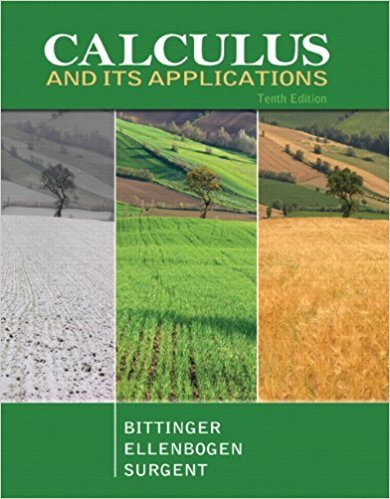×
×

# Solutions for Chapter 2.3: Graph Sketching: Asymptotes and Rational Functions## Full solutions for Calculus and Its Applications | 10th Edition

ISBN: 9780321694331Solutions for Chapter 2.3: Graph Sketching: Asymptotes and Rational Functions

Solutions for Chapter 2.3
4 5 0 257 Reviews
14
5
##### ISBN: 9780321694331

This expansive textbook survival guide covers the following chapters and their solutions. Since 89 problems in chapter 2.3: Graph Sketching: Asymptotes and Rational Functions have been answered, more than 25039 students have viewed full step-by-step solutions from this chapter. Calculus and Its Applications was written by and is associated to the ISBN: 9780321694331. Chapter 2.3: Graph Sketching: Asymptotes and Rational Functions includes 89 full step-by-step solutions. This textbook survival guide was created for the textbook: Calculus and Its Applications, edition: 10.

Key Calculus Terms and definitions covered in this textbook

If u = v and w = z , then u + w = v + z

• Combinations of n objects taken r at a time

There are nCr = n! r!1n - r2! such combinations,

• Coterminal angles

Two angles having the same initial side and the same terminal side

• Equivalent systems of equations

Systems of equations that have the same solution.

• Finite sequence

A function whose domain is the first n positive integers for some fixed integer n.

• Finite series

Sum of a finite number of terms.

• Frequency distribution

See Frequency table.

• Head minus tail (HMT) rule

An arrow with initial point (x1, y1 ) and terminal point (x2, y2) represents the vector <8x 2 - x 1, y2 - y19>

• Linear factorization theorem

A polynomial ƒ(x) of degree n > 0 has the factorization ƒ(x) = a(x1 - z1) 1x - i z 22 Á 1x - z n where the z1 are the zeros of ƒ

• NDER ƒ(a)

See Numerical derivative of ƒ at x = a.

• Obtuse triangle

A triangle in which one angle is greater than 90°.

• Order of an m x n matrix

The order of an m x n matrix is m x n.

• Permutation

An arrangement of elements of a set, in which order is important.

• Polar form of a complex number

See Trigonometric form of a complex number.

• Quartic function

A degree 4 polynomial function.

• Solve graphically

Use a graphical method, including use of a hand sketch or use of a grapher. When appropriate, the approximate solution should be confirmed algebraically

• Square matrix

A matrix whose number of rows equals the number of columns.

• Triangular form

A special form for a system of linear equations that facilitates finding the solution.

• Venn diagram

A visualization of the relationships among events within a sample space.

• Vertical component

See Component form of a vector.

×